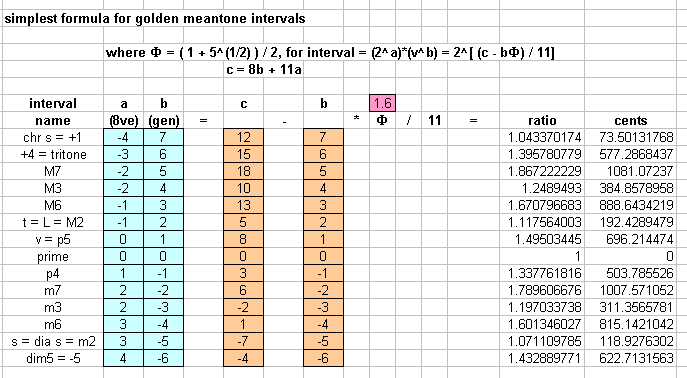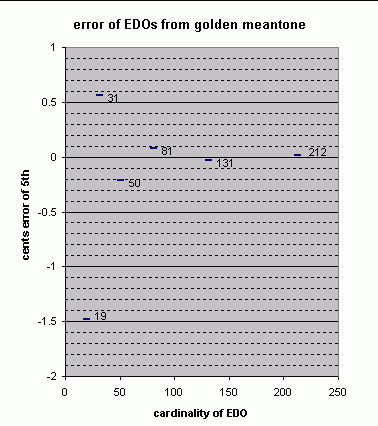# golden meantone

[Joe Monzo, with thanks to Tom Dourado and Gene Ward Smith.]

A tuning developed by Thorvald Kornerup and advocated by Jacques Dudon, this tuning is based on Phi, the golden mean (written with the Greek capital letter Φ "Phi") = (1 + 51/2) / 2 = ~1.61803398875

Phi is a unique constant. It is:

• the ratio of two quantities such that the smaller is to the greater, as the greater is to the sum of the greater and the smaller:
```where a < b :  b / a  =  (a+b) / b ;
```
• one larger than its own reciprocal; thus by definition: ( 1 / Φ ) = ( Φ - 1 ) .
• the mean of quotients of adjacent elements in a series.

(Kornerup's calculations were actually based on ( Φ - 1 ), which mathmaticians call φ "phi" in lower-case, and which Kornerup wrote as ω, the lower-case of the Greek letter omega. Also, closely related to this topic is that of Fibonacci numbers, which form the integer terms of fractions which approximate Φ and φ.)

This is a type of meantone composed of two different step sizes (designated L and s) such that 5L + 2s = one octave, as in many familiar diatonic scales. The "golden ratio" Φ comes into play in this tuning because it describes the ratio between log(s) and log(L) -- that is, the ratio between the perceived pitch-heights of s and L (and also of many other pairs of intervals in this tuning).

First we will set down the relationships for the most basic intervals, using only the data we already know here, and our knowledge of the usual number of meantone generators for the tone and semitone (L and s), then we will use algebra to derive the ratio for the generator, which is the tempered meantone version of the "perfect 5th", entirely in terms of the golden mean Φ, then we will use that value in calculating other intervals, including s and L.

Thus:

```Φ = golden ratio = (1 + 5(1/2) ) / 2

s = diatonic semitone =  t(1/Φ) = t(Φ-1) = 23/v5

t [= L] = tone = sΦ = v2/2

v = "5th" = t3 * s
```

So, t = sΦ is one suitable place to start.

```t = sΦ

v2     ( 23 )Φ
= ---  =  (--- )
2      ( v5 )

v2      23Φ
= ---  =  ----
2       v5Φ

Multiply both sides by v5Φ :

v(5Φ+2)
------    =    23Φ
2

Multiply both sides by 2 :

v(5Φ+2)  =  2(3Φ+1)

To solve for v, the exponent of 2 becomes the numerator of
a fraction, and the exponent of v becomes its denominator:

v  =   2[(3Φ+1)/(5Φ+2)]

```

And that exponent of 2 can be simplified still further. Gene Ward Smith wrote (Yahoo tuning-math group message 2507 (Mon Jan 7, 2002 7:31 pm):

Ratios of the sort (a+br)/(c+dr) define an algebraic number field, which can always be put into the form of a sum of rational numbers times powers of a single algebraic number r. In this case, that results in

This form of the algebraic numbers in the field Q(r) is unique, since {1, r} are a basis for a vector space over the rationals Q; hence we can determine if two elements of Q(r) are the same by putting them both into this form.

If we rearrange the exponent of 2 (in the ratio for v) so that it reads (1+3Φ)/(2+5Φ), and plug the values a=1, b=3, c=2, d=5, and Φ=r, into Gene's equation, the resulting answer is (-8+Φ)/-11, which can be simplified to (8-Φ)/11.

Thus, the golden meantone generator or "5th" "v", has the ratio of

2[ (8 - Φ) / 11 ] = ~1.49503444952678 = ~696.214474 cents,

and is composed of three Large (3L) plus one small note (s), i.e. (3L+s) = (~192.429*3) + (~118.928) = ~696.215 cents.

The golden meantone "5th" or generator is thus near the middle of the meantone spectrum, with an interval size only a tiny bit larger than the "optimal" 7/26-comma meantone (advocated by Woolhouse and Erlich) and a little smaller than 1/4-comma. (see the graph near the bottom of the meantone entry).

Expressed as a fraction-of-a-comma meantone, the golden meantone generator is nearly indistinguishable from that of 4/15-comma meantone:

```  --- prime-factor vector ---     ~ratio      ~cents
2      3     5

[        1/15   -1/15   4/15 ]   1.495039213	 696.2199903   4/15-comma meantone "5th"
- [    (8-Φ)/11    0      0]      1.49503445	 696.214474     golden meantone "5th"
---------------------------------
[(15Φ-109)/165  -1/15  4/15 ]   1.000003186   0.005516352 = ~1/181 cent = < 1 14mu
```

Golden meantone is also audibly indistinguishable from the 7/26-comma "optimal meantone" discovered independently by Woolhouse and Erlich:

```  --- prime-factor vector ---     ~ratio      ~cents
2      3     5

[   (8-Φ)/11    0     0   ]  1.49503445  696.214474    golden meantone "5th"
- [        1/13  -1/13  7/26]  1.494991593 696.164846    7/26-comma meantone "5th"
-----------------------------
[(93-13Φ)/143  1/13  -7/26]  1.000028667   0.049627981 = ~1/20 cent = ~2 12mus
```

Because Φ is such a special mathematical relationship, there are many pairs of intervals in golden meantone which have this relationship between their perceived sizes. Therefore, there are many ways to solve the equations to find out what "v" is in terms of Φ. Here are several others:

2[ (8Φ+1) / (13Φ+3) ], derived from v = t3 * s, a usual diatonic equation;

= 2[ (4Φ+3) / (7Φ+5) ], derived from m2 = #1Φ
(diatonic semitone = chromatic semitoneΦ ), which is (23/ v5) = (v7/ 24)Φ;

= 2[ (2Φ-1) / (3Φ-1) ], derived from p4 = m3Φ which is (2 / v) = (22 / v3)Φ;

= 2[ (3-Φ) / (4-Φ) ], derived from m6 = p4Φ, which is (23/ v4) = (2 / v)Φ ,

which all reduce to 2[(8-Φ)/11].

Any meantone interval can be designated in the form 2a * vb , where v is the generator (2[(8-Φ)/11] in this case), b is the number of generators needed to produce the octave-invariant form of that interval, and a is the amount of octave that must be added or subtracted to fit it into the reference octave.

The ratio of any golden meantone interval can be calculated from this notation very simply, with the formula 2[([ b * (8-Φ) ] / 11 ) + a ], which simplifies to 2[ (8b + 11a - bΦ) / 11 ]

Below is a table of the 14 intervals resulting from -6 to +7 generators, with their simplified golden meantone ratios. A fuller description of the most important ones follows.• As with all meantones, the golden meantone major-3rd is +4 generators minus 2 "8ves", or v4 / 22. This has the ratio 2[([4*(8-Φ)]/11)-2] = 2[(10-4Φ)/11] = ~1.2489493 = ~384.8578958 cents.
• The Large interval (L) is the whole-tone (IInd), +2 generators minus one octave = v2 / 2 , with the ratio 2[(5-2Φ)/11] = ~1.117564003 = ~192.4289479 cents.
• The small interval (s) is half the difference between 5 Large intervals and one octave, i.e. (8ve - 5L) / 2 , and is the minor-2nd or "flat second" (bII), -5 generators plus 3 "8ves" = 23 / v5, with the ratio 2[(5Φ-7)/11] = ~1.071109785. That is s = ~118.9276302 cents. This interval functions as the diatonic semitone in this tuning.
• The chromatic semitone or "augmented prime" (aug1, #1, +1) is represented in meantone as +7 generators minus 4 octaves, or v7 / 24 . In golden meantone its ratio is 2[(12-7Φ)/11] = ~1.043370174 = ~73.50131768 cents.

EDOs which approximate golden meantone to increasing degrees of accuracy are:

 ```EDO degrees cents ~cents error from of "5th" golden meantone "5th" 12 7 700 +3.785526045 19 11 ~694.7368421 -1.477631849 31 18 ~696.7741935 +0.559719594 50 29 696 -0.214473955 81 47 ~696.2962963 +0.081822342 131 76 ~696.1832061 -0.031267848 212 123 ~696.2264151 +0.01194114 555 322 ~696.2162162 +0.001742262 898 521 ~696.2138085 -0.000665491 (12edo lacks many of the features of golden meantone, and is shown only for the sake of comparison.) ```. . . . . . . . .

The tonalsoft.com website is almost entirely the work of one person: me, Joe Monzo. Please reward me for my knowledge and effort by selecting the highest level of financial support that you can afford. Thank you.

 support level patron: \$50 USD savior: \$100 USD angel of tuning: \$500 USD microtonal god: \$1000 USD friend: \$25 USD donor: \$5 USD